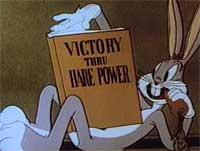#Difference Between Similar Terms and Objects

# Difference Between Pressure and ForcePressure vs Force

Force

Force is the pull or push on an object which can change the velocity of the object on which it is applied. We can say that if force is applied on an object from one direction and there is no opposing force, then the object will move. If it’s already moving, it will either get faster or slower depending upon the direction of the applied force.

This can be simplified by taking the example of a moving ball. When somebody kicks a ball, that is, applies force on it in a particular direction, the ball moves. If the ball is already in motion and somebody kicks it harder in the direction of the motion, then the velocity of the ball increases.

This is true only in the case when force is applied from one direction without much opposition. When there is force acting in the opposite direction, then the object will move accordingly. If the opposite force is stronger, the ball will move in reverse. Or if the force in each direction is equal, then the object comes to a halt. Alternatively, the motion will be in the resultant direction. This can be explained by giving the example of a man sitting in a chair. The force applied by your body on the chair is equal to the force of the chair pushing back your body’s force. Thus a person remains in the chair with zero resultant motion.

Force is represented by magnitude and direction. It is a vector quantity. It is measured by Newton’s law. F=m X a. “F” stands for “force,” “m” stands for “mass,” and “a” stands for “acceleration.” “Acceleration” is defined as “the rate of change of velocity.”

The great physician Isaac Newton also described force in his first law of motion. According to this, in order to change the direction of the motion of an object, a force has to be applied on the body.

Pressure

Pressure can be explained as force applied on a unit area. The formula used to find out pressure is P=F/A. “P” stands for “pressure,” “F” stands for “force,” and “A” stands for “area.”

When the force is applied over a large area, then the pressure developed is lower as compared to that developed on a smaller area.

The unit of pressure is Pascals’law. Pressure is a scalar quantity as it is not dependent upon the direction; it is only dependent upon magnitude.

Summary:

1.The unit of force in the metric system is Newton’s law and in the US the unit is pound. The unit of pressure is Pascal’s law which can also be called Newton’s law per square meter or pound per square inch.
2.Pressure is explained as the force being spread over a particular area of an object on which it is acting upon; whereas force can be explained as the energy applied to an object in order to change the object’s direction of motion.
3.Force if applied from one direction can change the object’s velocity. Pressure does not change the velocity of the object.
4.Force is vector quantity, that is, it depends upon the magnitude of an object and its direction both; whereas pressure depends only upon magnitude. It is a scalar quantity.

Latest posts by Nimisha Kaushik (see all)

### Search DifferenceBetween.net :

Custom Search

Help us improve. Rate this post!(7 votes, average: 4.14 out of 5)Loading...Email This Post : If you like this article or our site. Please spread the word. Share it with your friends/family.